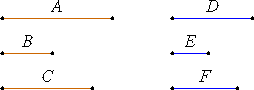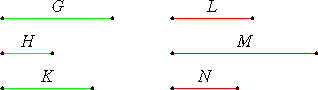# Definition 17

A ratio ex aequali arises when, there being several magnitudes and another set equal to them in multitude which taken two and two are in the same proportion, the first is to the last among the first magnitudes as the first is to the last among the second magnitudes. Or, in other words, it means taking the extreme terms by virtue of the removal of the intermediate terms.

# Definition 18

A perturbed proportion arises when, there being three magnitudes and another set equal to them in multitude, antecedent is to consequent among the first magnitudes as antecedent is to consequent among the second magnitudes, while, the consequent is to a third among the first magnitudes as a third is to the antecedent among the second magnitudes.

## Guide

 If A : B = D : E, and B : C = E : F, then as shown in proposition V.22, ex aequali, A : C = D : F. In Book VII ratios of three terms are used in proposition VI.33. In that proposition, a certain proportion A : B : C = E : F : G is shown. This multiterm proportion may be interpreted as an abbreviation for two proportions A : B = E : F and B : C = F : G. Then it follows ex aequali that A : C = E : G.However, if G : H = M : N, and H : K = L : M, then a perturbed proportion holds as shown in proposition V.23, namely, G : K = L : N.